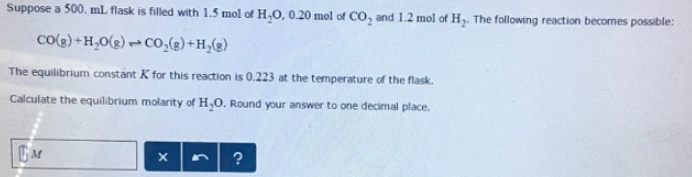# Problem: Suppose a 500. mL flask is filled with 1.5 mol of H 2O, 0.20 mol of CO2 and 1.2 mol of H2. The following reaction becomes possible: CO (g) + H2O (g) ⇌ CO2 (g) + H2 (g) The equilibrium constant K for this reaction is 0.223 at the temperature of the flask. Calculate the equilibrium molarity of H2O.

###### FREE Expert Solution
85% (318 ratings)###### Problem Details

Suppose a 500. mL flask is filled with 1.5 mol of H 2O, 0.20 mol of CO2 and 1.2 mol of H2. The following reaction becomes possible:

CO (g) + H2O (g) ⇌ CO2 (g) + H2 (g)

The equilibrium constant K for this reaction is 0.223 at the temperature of the flask.

Calculate the equilibrium molarity of H2O.